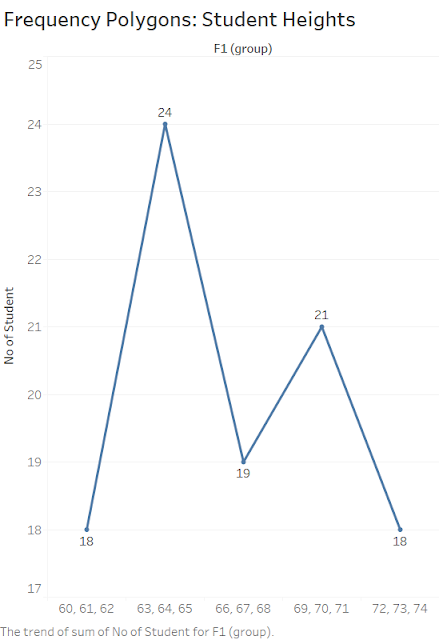# DataGenX

My e-Notes about DataScience, Machine Learning, Python, Data Analytics, DataStage, DWH and ETL Concepts

## Tuesday, 30 July 2019

Starting from the point where we left off - Frequency Distribution #1 - #UnlockStats
Below is the table for 100 students and their heights categories -
 Height (in) No of Students 60-62 5 63-65 18 66-68 38 69-71 31 72-74 8 total 100#### Histogram:

It consists of a set of rectangles having based on a horizontal axis with center at the class mark and width equal to the class intervals size and length proportional to class frequency.

The histogram shows how the data is distributed, In our example, the width is 3 of each category and left-skewed. Most of the data is left side of the histogram

####Frequency Polygon:

A Frequency Polygon is line graph of the class frequencies plotted against class marks = ( UCL + LCL ) / 2
It can be obtained by connecting the midpoint of the tops of the rectangles in the histogram.#### Box Plots:

A box plot shows a box which contains the middle 50% of data values, It also shows two whiskers that extend from the box to maximum and minimum value.

#### Relative Frequency Distribution:

The Relative Frequency of a class is the frequency of the class divided by total frequency of all the classes (total no of data points) and expressed in percentage.
 Height (in) Relative Frequency Distribution (%) 60-62 5 63-65 18 66-68 38 69-71 31 72-74 8

#### Cumulative Frequency Distribution:

The total frequency of all values less than the upper-class boundary of a given class interval is called the cumulative frequency up to and including that class interval.
 Height (in) No of Students Cum. Freq. Distribution 60-62 ( <=62) 5 5 63-65 (<=65) 18 5+18 = 23 66-68 (<=68) 38 23 + 38 = 16 69-71 (<=71) 31 61 + 31 = 92 72-74 (<=74) 8 92 + 8 = 100

A line plot between Upper-Class Boundary and Cum. Frequency is called Cum Freq Distribution polygon or ogive.

#### Cumulative Relative Frequency Distribution:

 Height (in) No of Students Cum. Rel. Freq. Distribution (%) <=62 5 5 <=65 18 23 <=68 38 16 <=71 31 92 <=74 8 100

23% of the students have less than or equal to 65 inches.

#### Types of Frequency Curves:

a. Symmetrical or bell curves are characterized by the fact that observations equidistance from the central maximum has the same frequency.
b. Curves that have tails to the left are said to be skewed to the left.
c. Curves that have tails to the right are said to be skewed to the right.
d. Curves that have approx equal frequencies across their values are said to be uniformly distributed.
e. J-shaped or reverse J-shaped frequency curve the maximum occurs at one end or the other.
f. A U-shaped curve has maxima at both end and minimum in between.
g. A bimodal frequency curve has two maxima.
h. A multimodal frequency curve has more than 2 maxima.

Like the below page to get the update